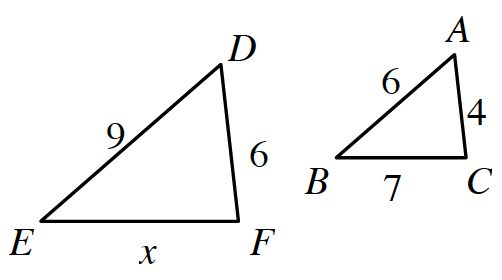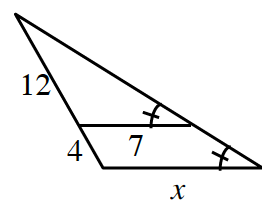### Home > CC2MN > Chapter 11 > Lesson 11.1.5 > Problem11-67

11-67.

First decide if the triangles below are similar. Then, if they are similar, use the information in each diagram to solve for $x$.

1.If only two pair of corresponding sides have equal ratios, can you conclude that the triangles are similar?

1. Careful!The two triangles are similar (by $\text{AA}\sim$).

Draw two separate triangles, then write a proportion and solve for $x$.

$\frac{12}{7}=\frac{16}{x}$